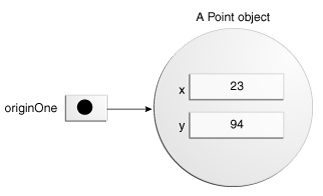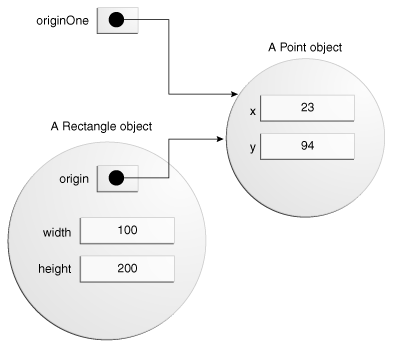# # 创建对象

``````Point originOne = new Point(23, 94);
Rectangle rectOne = new Rectangle(originOne, 100, 200);
Rectangle rectTwo = new Rectangle(50, 100);
``````
1
2
3

• 声明：等号左边的代码都是将变量名称与对象类型相关联的变量声明。
• 实例化：new 关键字是 Java 创建对象的操作符。
• 初始化：new 运算符后跟一个构造函数的调用，该构造函数初始化新对象。

## # 实例化一个类

`new` 通过一个新的对象分配内存，并返回到内存的引用实例化一个类。在 new 的操作符后还调用对象的构造函数。

TIP

`new` 操作符需要一个单一的，后缀的参数：一个构造函数的调用。构造函数的名称提供要实例化的类的名称。

new 操作符反回到它创建的对象的引用。此引用通常分配给适当类型的变量，如：

``````int height = new Rectangle().height;
``````
1

## # 初始化对象

``````public class Point {
public int x = 0;
public int y = 0;
//constructor
public Point(int a, int b) {
x = a;
y = b;
}
}
``````
1
2
3
4
5
6
7
8
9

``````Point originOne = new Point(23, 94);
``````
1``````public class Rectangle {
public int width = 0;
public int height = 0;
public Point origin;

// four constructors
public Rectangle() {
origin = new Point(0, 0);
}
public Rectangle(Point p) {
origin = p;
}
public Rectangle(int w, int h) {
origin = new Point(0, 0);
width = w;
height = h;
}
public Rectangle(Point p, int w, int h) {
origin = p;
width = w;
height = h;
}

// a method for moving the rectangle
public void move(int x, int y) {
origin.x = x;
origin.y = y;
}

// a method for computing the area of the rectangle
public int getArea() {
return width * height;
}
}
``````
1
2
3
4
5
6
7
8
9
10
11
12
13
14
15
16
17
18
19
20
21
22
23
24
25
26
27
28
29
30
31
32
33
34

``````Rectangle rectOne = new Rectangle(originOne, 100, 200);
``````
1``````Rectangle rectTwo = new Rectangle(50, 100);
``````
1

``````Rectangle rectTwo = new Rectangle();
``````
1